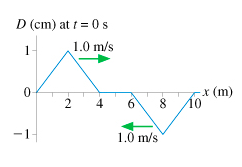# Problem: The figure is a snapshot graph at t = 0s of two waves approaching each other at 1.0 m/s. Draw six snapshot graphs, stacked vertically, showing the string at 1s intervals from t = 1s to t = 6s.t = 1st = 2st = 3st = 4st = 5st = 6s

###### FREE Expert Solution

In this problem, we are dealing with the superposition of waves. We have two waves approaching each other.

92% (473 ratings)###### Problem Details

The figure is a snapshot graph at t = 0s of two waves approaching each other at 1.0 m/s. Draw six snapshot graphs, stacked vertically, showing the string at 1s intervals from t = 1s to t = 6s.t = 1s

t = 2s

t = 3s

t = 4s

t = 5s

t = 6s

Frequently Asked Questions

What scientific concept do you need to know in order to solve this problem?

Our tutors have indicated that to solve this problem you will need to apply the Waves on a String concept. You can view video lessons to learn Waves on a String. Or if you need more Waves on a String practice, you can also practice Waves on a String practice problems.

What professor is this problem relevant for?

Based on our data, we think this problem is relevant for Professor Mechanica's class at NYU TANDON.